Win up to 100% scholarship on Aakash BYJU'S JEE/NEET courses with ABNAT Win up to 100% scholarship on Aakash BYJU'S JEE/NEET courses with ABNAT

# Activation Energy

Activation energy is often studied under physical chemistry and it is a very important concept related to chemical kinetics in JEE. The term Activation Energy was first used by a Swedish scientist named Svante Arrhenius in the year 1889. Today, we learn about this concept as it helps us understand the energy requirements for a chemical reaction, which further gives us control over our actions and the environment.

Almost all the chemical reactions require some activation energy to go forward. Therefore, it is important for students to know what activation energy is. We shall learn more about this concept in this lesson.

## Activation Energy Definition

Activation energy is defined as the minimum amount of extra energy required by a reacting molecule to get converted into a product. It can also be described as the minimum amount of energy needed to activate or energise molecules or atoms so that they can undergo a chemical reaction or transformation.

## What Is the SI Unit of Activation Energy?

Activation energy is denoted by Ea. It is usually measured in joules (J) and or kilojoules per mole (kJ/mol) or kilocalories per mole (kcal/mol).

## Factors Affecting Activation Energy

Activation energy depends on two factors.

1. Nature of Reactants

In the case of ionic reactant, the value of (Ea) will be low because there is an attraction between reacting species. While in the case of covalent reactant the value of Ea will be high because energy is required to break the older bonds.

2. Effect of Catalyst

Positive catalyst provides such an alternate path in which the value of Ea will be low, while the negative catalyst provides such an alternate path in which the value of Ea will be high.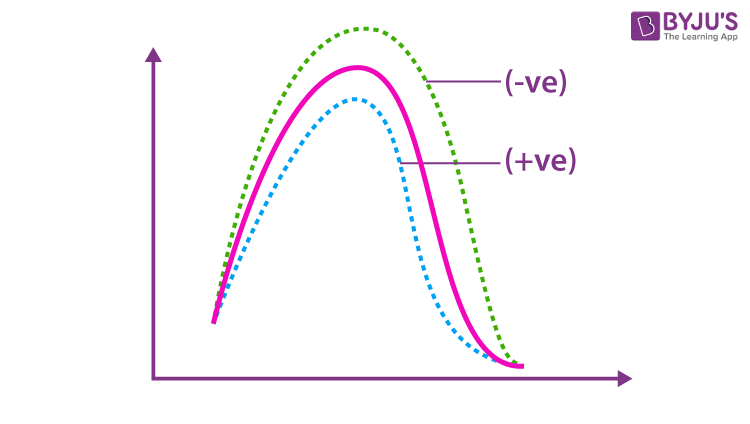Note: Activation energy does not depend upon the temperature, pressure, volume, concentration, or coefficients of reactant.

## Activation Energy Examples

### Exothermic Reaction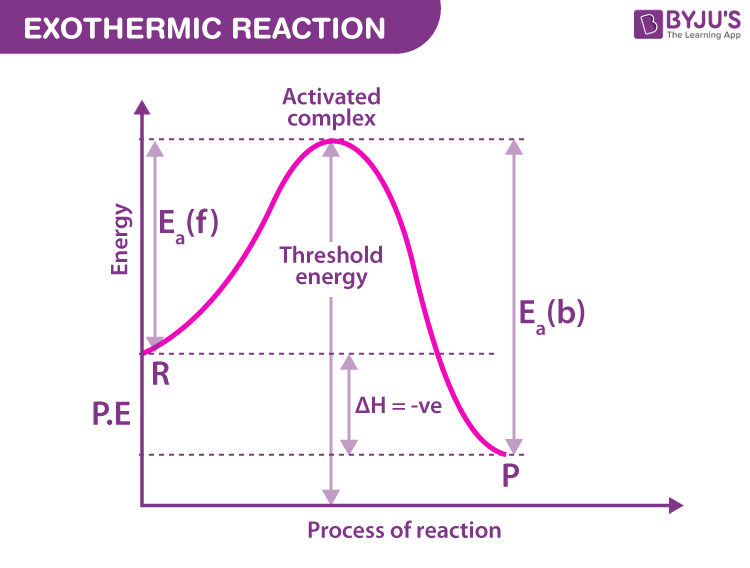Activation energy for Forward reaction (Ea)f

Activation energy for Forward reaction (Ea)b

(Ea)f < (Ea)b

(ROR)f > (ROR)b

△H = (Ea)f – (Ea)b

△H = -ve value

(Ea)f = △H Only one can be possible for Exothermic Reaction

(Ea)f > △H

(Ea)f < △H

Endothermic Reaction

(Ea)f > (Ea)b

(ROR)f < (ROR)b

△H = (Ea)b – (Ea)f

△H = +ve value

(Ea)f > △H (Always )…………universal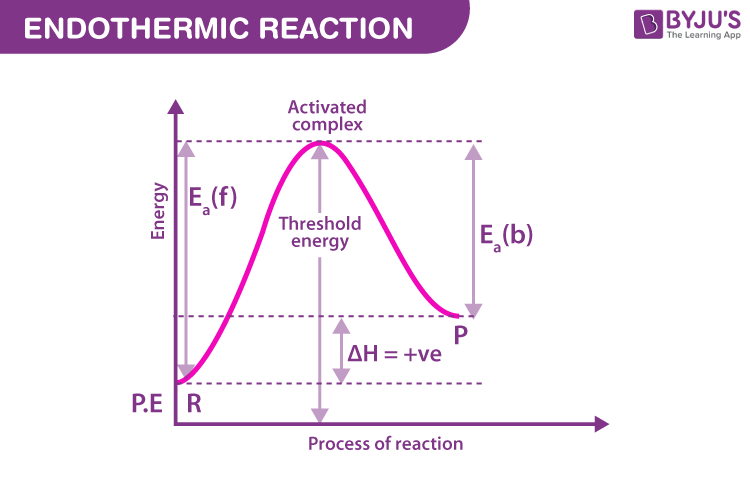## Catalysts

A catalyst is a chemical substance that either increases or decreases the rate of a chemical reaction. In the case of activation energy, a catalyst lowers it. However, the energies of the original reactants remain the same. A catalyst only alters the activation energy.

### Types of Catalysts

Positive Catalysts

A catalyst that helps to increase the rate of reaction or which support the reaction to carry out quickly is called a positive catalyst. Such catalyst decreases activation energy by accepting a smaller path, so the rate of reaction is increased.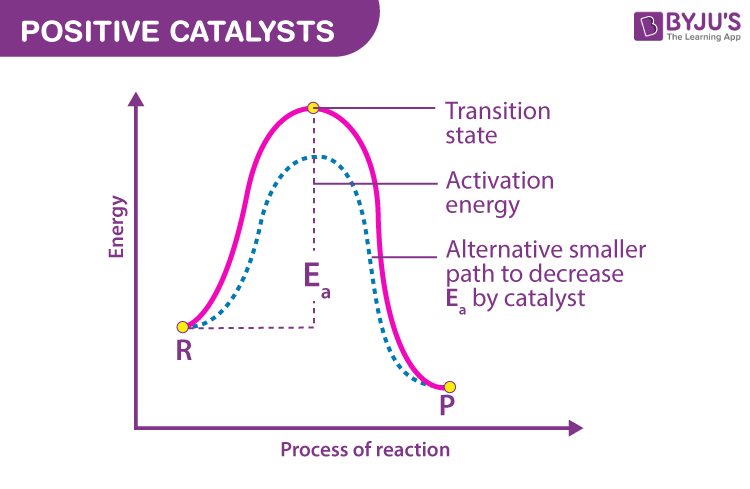Negative Catalysts (Inhibitors)

A catalyst that decreases or retards or helps in slowing down the rate of reaction is called a negative catalyst.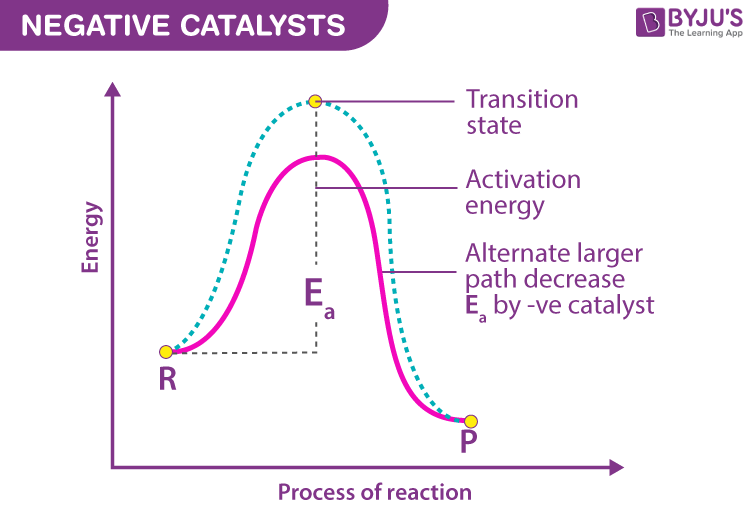It is because a negative catalyst increases activation energy by taking a longer alternative path.

### Points to Remember

Forward Reaction: Those reactions in which the product formed are produced from reactants

Backward Reaction: These are those reactions when reaction goes from products to reactants.

## Activated Complex

When reactant molecules collide with each other at its highest energy point, an intermediate is formed which remains in equilibrium with the main reactant. If this intermediate complex has an energy equal to or greater than the Threshold Energy, it will be converted into a product.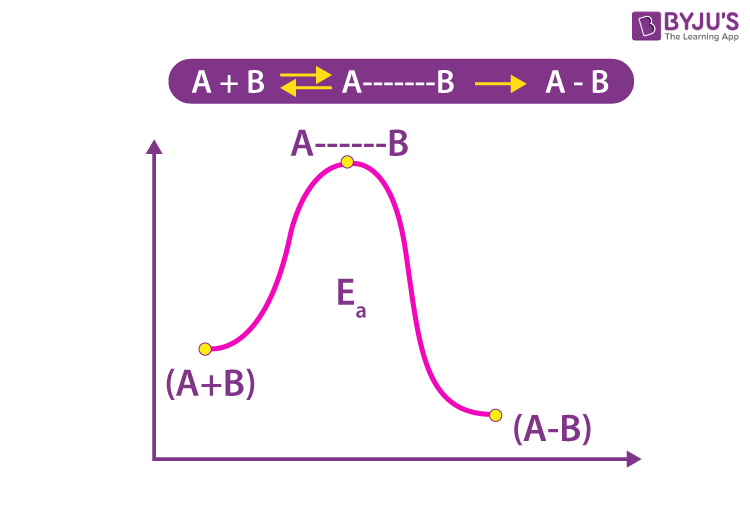## Activation Energy Formula

The formula used to find the value of Activation Energy, Ea is;

K = Ae-Ea/RT

Where

K = Rate Constant

A = Arrhenius Constant

Ea = Activation Energy

R = Gas constant = 8.34J/K/mol

=8.314/1000 KJ/K/mol

= 2 cal/K/mol

= 0.0821 lit atm/K/mol

K = Ae-Ea/RT

Taking log on both sides

ln K = ln A – (Ea /RT)ln e

2.303 log K = 2.303 log A – Ea/RT

log K = log A – Ea /2.303RT

## Solved Examples Related to Activation Energy

1. The rate constant of a 1st order reaction increases from 3 × 10-2 to 8 × 10-2 when the temperature changes from 310K to 330K. Calculate the activation energy (Ea).

Solution:

Given k2 = 8 × 10-2, k1 = 3 × 10-2, T310KT2 330K

$$\begin{array}{l}log \frac{k_{1}}{k_{2}} = \frac{E_{a}}{2.303 R}\left ( \frac{1}{T_{1}}-\frac{1}{T_{2}} \right )\end{array}$$
$$\begin{array}{l}log\frac{8\times 10^{-2}}{3\times 10^{-2}} = \frac{E_{a}}{2.303 R}\left ( \frac{1}{310} -\frac{1}{330}\right )\end{array}$$
$$\begin{array}{l}log 2.66 =\frac{E_{a}}{2.303 R}(1.95503\times 10^{-4})\end{array}$$
$$\begin{array}{l}0.4249 = \frac{E_{a}}{2.303\times 8.314}\times (1.95503\times 10^{-04})\end{array}$$
$$\begin{array}{l}0.4249 = \frac{E_{a}}{19.147}\times (1.95503\times 10^{-04})\end{array}$$
$$\begin{array}{l}0.4249 = 1.02106\times 10^{-5}\times E_{a}\end{array}$$

Ea = 41,613.62 J/mole

= 41.614 kJ/mol

2. The rate constant of the first-order reaction raises from 3 X 10-2 to 5×10-2 when the temperature changes from 300K to 310K. Calculate the activation energy.

Solution:

Given k2 = 3 × 10-2, k1 = 5 × 10-2, T= 300K, T2 = 310K

$$\begin{array}{l}log \frac{k_{1}}{k_{2}} = \frac{E_{a}}{2.303 R}\left ( \frac{1}{T_{1}}-\frac{1}{T_{2}} \right )\end{array}$$
$$\begin{array}{l}log\frac{5\times 10^{-2}}{3\times 10^{-2}} = \frac{E_{a}}{2.303 R}\left ( \frac{1}{300} -\frac{1}{310}\right )\end{array}$$
$$\begin{array}{l}log 1.66 =\frac{E_{a}}{2.303 R}(1.07526\times 10^{-04})\end{array}$$
$$\begin{array}{l}0.2218 = \frac{E_{a}}{2.303\times 8.314}\times (1.07526\times 10^{-04})\end{array}$$
$$\begin{array}{l}0.2218 = \frac{E_{a}}{19.147}\times (1.07526\times 10^{-04})\end{array}$$
$$\begin{array}{l}0.2218 = 5.6158\times 10^{-6}\times E_{a}\end{array}$$

Ea = 39,495.7 J/mole

= 39.495 kJ/mol

3.  The first order reaction has rate constant of 2.0×10-2 and 6.0×10-2 at 00C and 300C. Calculate the activation energy of the reaction.

Solution:

Given k2 = 6 × 10-2, k1 = 2 × 10-2, T= 273K, T2 = 303K

$$\begin{array}{l}log \frac{k_{1}}{k_{2}} = \frac{E_{a}}{2.303 R}\left ( \frac{1}{T_{1}}-\frac{1}{T_{2}} \right )\end{array}$$
$$\begin{array}{l}log\frac{6\times 10^{-2}}{2\times 10^{-2}} = \frac{E_{a}}{2.303 R}\left ( \frac{1}{273} -\frac{1}{303}\right )\end{array}$$
$$\begin{array}{l}log 3 =\frac{E_{a}}{2.303 R}(3.6267\times 10^{-04})\end{array}$$
$$\begin{array}{l}0.4771 = \frac{E_{a}}{2.303\times 8.314}\times (3.6267\times 10^{-04})\end{array}$$
$$\begin{array}{l}0.4771 = \frac{E_{a}}{19.147}\times (3.6267\times 10^{-04})\end{array}$$
$$\begin{array}{l}0.4771 = 1.8941\times 10^{-5}\times E_{a}\end{array}$$

Ea = 25,188.74 J/mole

= 25.188 kJ/mol

## Frequently Asked Questions – FAQs

Q1

### What is the meaning of high activation energy?

High activation energy means high energy required to have a successful collision between the particles, such as combustion. Even though combustion is an exothermic reaction, it requires heat because of the high activation energy. The particle gains enough energy from heat to overcome the barrier of activation energy.
Q2

### What is the difference between activation energy and energy?

Energy is the capacity to do some work, whereas activation energy is the energy needed to form the activated complex during the chemical reaction.
Q3

### What is a negative catalyst?

A catalyst that decreases the reaction rate is called a negative catalyst. The negative catalyst increases the activation energy of the reaction.
Q4

### Does activation energy depend on temperature, pressure and other variables?

No, activation energy does not depend on factors like temperature or pressure.
Q5

### What is the SI unit of activation energy?

The SI unit of activation energy is KJ/mol
Test your Knowledge on activation energy